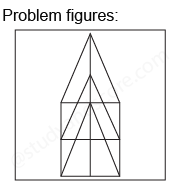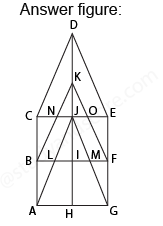## Practice Test Discussion

Q)

How many triangles and parallelograms are there in the following figure?• ExplanationAfter labelling the figure, we get the following,

• Simplest triangles KJN, KJO, CNB, OEF, JIL, JIM, BLA and MFG (8)
• Triangles with two components each CDJ, EDJ, NKO, JLM, JAH, and JGH (6)
• Triangles with three components each BKI, FKI, CJA, and EJG (4)
• Triangles with four components each CDE and AJG (2) Triangle with six components is BKT (1)

Total number of triangles in the figure is 8 + 6 + 4 + 2 + 1 = 21

Also we need to count the number of parallelograms in this figure,

• Simplest parallelograms NJLB and JOFM (2)
• Parallelograms with two components each CDKB, DEFK, BIHA, and IFGH (4)
• Prallelograms with three components each BKJA, KFGJ, CJIB, and JEFI (4)
• Parallelogram composed of four components is BFGA (1)
• Parallelogram composed of six components is CEFB (1)
• Parallelogram composed of ten components is CEGA (1)

Total number of parallelograms in the figure is 2 + 4 + 4 + 1 + 4 + 1+ 1 =17

Hence option A is correct.

- Share with your friends! -